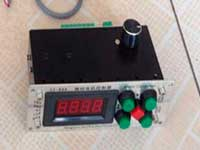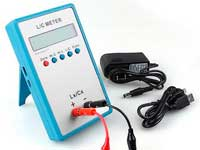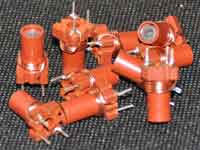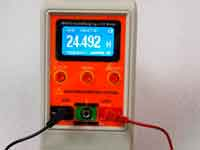Multilayer PCB inductor calculation - 4.0 out of 5 based on 4 votes
Rating 4.00 (4 Votes)

Multilayer PCB inductor calculationMulti-layer coil on a PCB is needed for those designers who want to create a miniature device with the use of inductance sufficiently high value. Single-layer coil on a PCB with inductance more that 10 µh usually has a relatively large size. To solve this problem will help the fabrication of multilayer printed coils. Such coils are used as various sensors, elements of radio frequency filters, etc. However, one should not forget that they have a relatively low quality factor, that is a natural payment for miniaturization. But this is in comparison with wire coils. If we compare with the SMD coils, multilayer printed coils have the best indicators of the Q-factor and smaller the self-capacitance.

The calculation of the multi-layer printed coils is implemented in the plugin multi_PCB and based on research of Jonsenser Zhao – the Chinese engineer. The plugin is not included in the main archive of Coil32 and available for free download from the Plugin Manager.

The total inductance of two  interrelated coils is determined by the formula:where:

• Ls - the total inductance;
• L1, L2 - self-inductance of the first and second coils;
• M - mutual inductance;

If the coils in each layer are identical and are included in phase, then the formula takes the following form:where:

• L0 -  the self-inductance of one of the coils;
• Kc - the coupling factor;

The total inductance of multi-layer printed coil we can compute as the sum of the inductances of each layer plus the mutual induction of each layer with all other layers. We have already applied this idea to the wire multilayer coil calculation. Only there, this calculation was done for each individual loop, as in this case – for each individual layer. For multi-layer printed coil with identical layer the calculation formula is as follows:n - the number of layers of the coil;

How to determine the inductance of one of the spirals in each layer we know. The problem is to determine the value of the coupling factor Kc. Jonsenser Zhao have solved this problem experimentally. He had made a number of multilayer printed coils with number of turns between 5 and 20 and the distance between adjacent layers from 0.75 to 2 mm. From the measurements was derived an empirical formula for computing coefficient of mutual induction between two layers of a multilayer printed coil:where:

Kc -  the coupling factor;
N - number of turns in the layer;
X - the distance between the layers in mm;

References:

Сomments from anonymous guests are enabled with moderation.

Coil Winding MachineUS \$187.53
The system consists of individual components including a controller, a stepping motor, a foot switch, power adapter and output shaft. This design makes it very easy for you to integrate the system into your own project.

LC Meter LC200AUS \$37.99
Measuring Capacitance Range:
0.01 pF - 100mF
Measuring Inductance Range:
0.001 uH - 100H
Measurement accuracy:1%.

RF InductorsUS \$6.43
Variable Ferrite Core Inductor coil set 3.5Turns 25-100MHZ

Auto Ranging LCR MeterUS \$63.07
Meter can measure 0.00pF to 100.00mF capacitance, 0.000uH to 100.00H inductance and 0.00? to 10.000M? resistance. Very suitable for small value capacitor and inductor measurement.